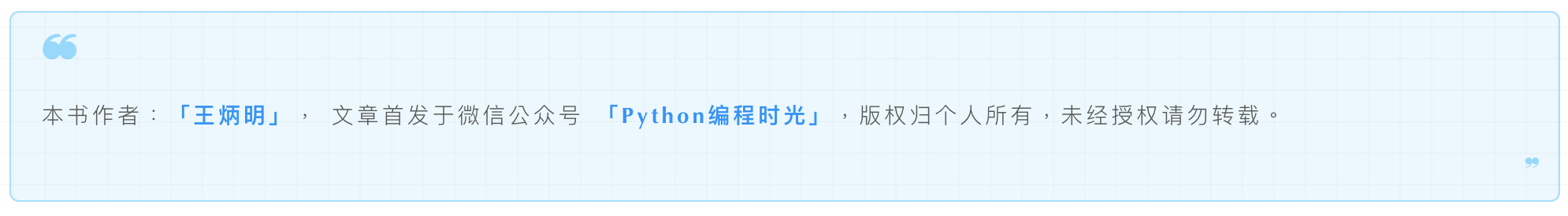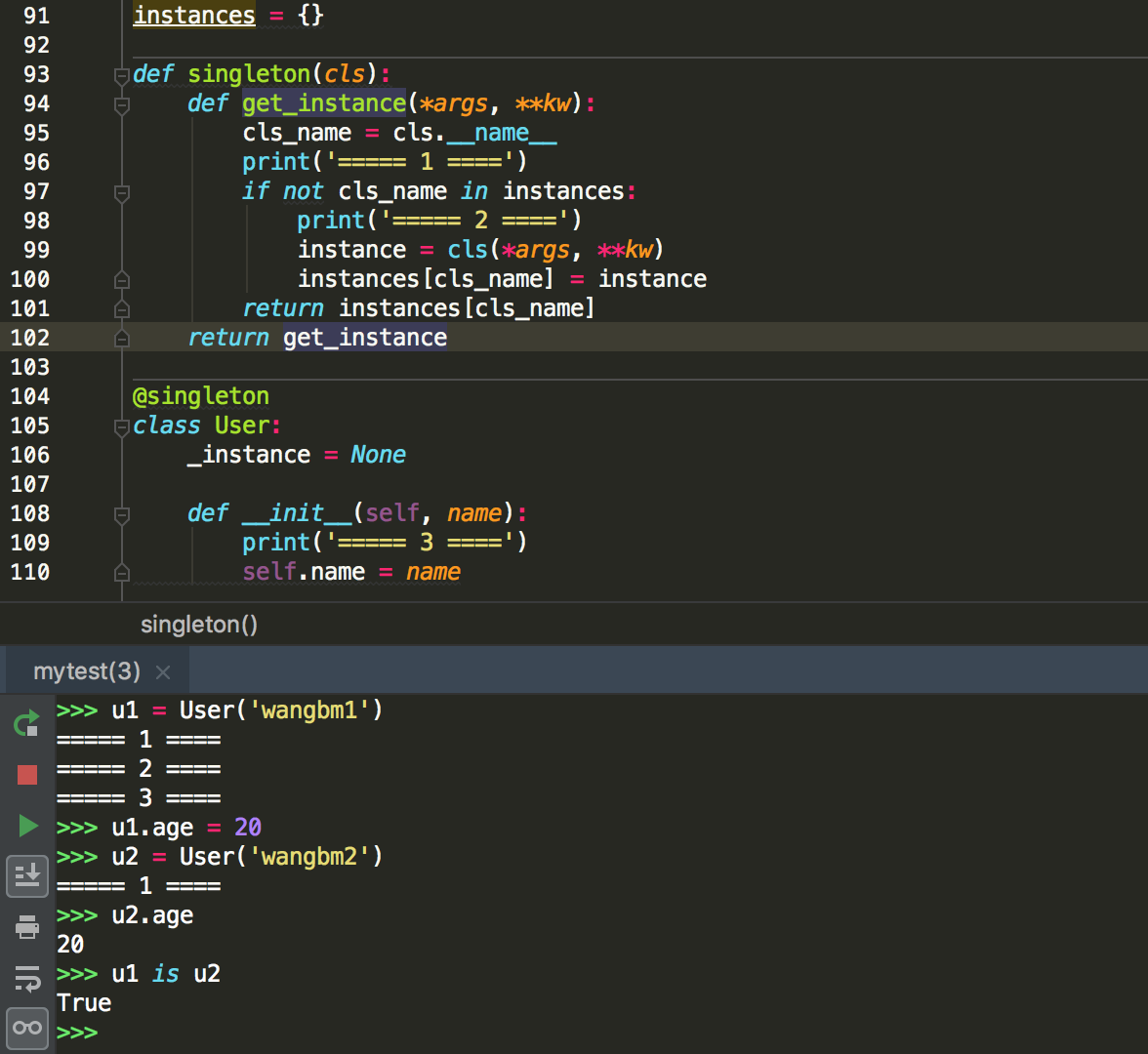# 4.1 精通装饰器八大用法¶## Hello，装饰器¶

• 先定义一个装饰器（帽子）

• 再定义你的业务函数或者类（人）

• 最后把这装饰器（帽子）扣在这个函数（人）头上

```def decorator(func):
def wrapper(*args, **kw):
return func()
return wrapper

@decorator
def function():
print("hello, decorator")
```

• 更加优雅，代码结构更加清晰

• 将实现特定的功能代码封装成装饰器，提高代码复用率，增强代码可读性

## 入门：日志打印器¶

• 在函数执行前，先打印一行日志告知一下主人，我要执行函数了。

• 在函数执行完，也不能拍拍屁股就走人了，咱可是有礼貌的代码，再打印一行日志告知下主人，我执行完啦。

```# 这是装饰器函数，参数 func 是被装饰的函数
def logger(func):
def wrapper(*args, **kw):
print('主人，我准备开始执行：{} 函数了:'.format(func.__name__))

# 真正执行的是这行。
func(*args, **kw)

print('主人，我执行完啦。')
return wrapper
```

```@logger
print('{} + {} = {}'.format(x, y, x+y))
```

```add(200, 50)
```

```主人，我准备开始执行：add 函数了:
200 + 50 = 250

```

## 入门：时间计时器¶

```# 这是装饰函数
def timer(func):
def wrapper(*args, **kw):
t1=time.time()
# 这是函数真正执行的地方
func(*args, **kw)
t2=time.time()

# 计算下时长
cost_time = t2-t1
print("花费时间：{}秒".format(cost_time))
return wrapper
```

```import time

@timer
def want_sleep(sleep_time):
time.sleep(sleep_time)

want_sleep(10)
```

```花费时间：10.0073800086975098秒
```

## 进阶：带参数的函数装饰器¶

```@periodic_task(spacing=60)
def send_mail():
pass

def ntp()
pass
```

```# 小明，中国人
@say_hello("china")
def xiaoming():
pass

# jack，美国人
@say_hello("america")
def jack():
pass
```

```def say_hello(contry):
def wrapper(func):
def deco(*args, **kwargs):
if contry == "china":
print("你好!")
elif contry == "america":
print('hello.')
else:
return

# 真正执行函数的地方
func(*args, **kwargs)
return deco
return wrapper
```

```xiaoming()
print("------------")
jack()
```

```你好!
------------
hello.
```

## 高阶：不带参数的类装饰器¶

```class logger(object):
def __init__(self, func):
self.func = func

def __call__(self, *args, **kwargs):
print("[INFO]: the function {func}() is running..."\
.format(func=self.func.__name__))
return self.func(*args, **kwargs)

@logger
def say(something):
print("say {}!".format(something))

say("hello")
```

```[INFO]: the function say() is running...
say hello!
```

## 高阶：带参数的类装饰器¶

`__init__` ：不再接收被装饰函数，而是接收传入参数。 `__call__` ：接收被装饰函数，实现装饰逻辑。

```class logger(object):
def __init__(self, level='INFO'):
self.level = level

def __call__(self, func): # 接受函数
def wrapper(*args, **kwargs):
print("[{level}]: the function {func}() is running..."\
.format(level=self.level, func=func.__name__))
func(*args, **kwargs)
return wrapper  #返回函数

@logger(level='WARNING')
def say(something):
print("say {}!".format(something))

say("hello")
```

```[WARNING]: the function say() is running...
say hello!
```

## 使用偏函数与类实现装饰器¶

```import time
import functools

class DelayFunc:
def __init__(self,  duration, func):
self.duration = duration
self.func = func

def __call__(self, *args, **kwargs):
print(f'Wait for {self.duration} seconds...')
time.sleep(self.duration)
return self.func(*args, **kwargs)

def eager_call(self, *args, **kwargs):
print('Call without delay')
return self.func(*args, **kwargs)

def delay(duration):
"""
装饰器：推迟某个函数的执行。
同时提供 .eager_call 方法立即执行
"""
# 此处为了避免定义额外函数，
# 直接使用 functools.partial 帮助构造 DelayFunc 实例
return functools.partial(DelayFunc, duration)
```

```@delay(duration=2)
return a+b
```

```>>> add    # 可见 add 变成了 Delay 的实例
<__main__.DelayFunc object at 0x107bd0be0>
>>>
Wait for 2 seconds...
8
>>>
```

## 如何写能装饰类的装饰器？¶

```instances = {}

def singleton(cls):
def get_instance(*args, **kw):
cls_name = cls.__name__
print('===== 1 ====')
if not cls_name in instances:
print('===== 2 ====')
instance = cls(*args, **kw)
instances[cls_name] = instance
return instances[cls_name]
return get_instance

@singleton
class User:
_instance = None

def __init__(self, name):
print('===== 3 ====')
self.name = name
```## wraps 装饰器有啥用？¶

```def wrapper(func):
def inner_function():
pass
return inner_function

@wrapper
def wrapped():
pass

print(wrapped.__name__)
#inner_function
```

```def wrapper(func):
def inner_function():
pass
return inner_function

def wrapped():
pass

print(wrapper(wrapped).__name__)
#inner_function
```

```from functools import wraps

def wrapper(func):
@wraps(func)
def inner_function():
pass
return inner_function

@wrapper
def wrapped():
pass

print(wrapped.__name__)
# wrapped
```

```def wraps(wrapped,
assigned = WRAPPER_ASSIGNMENTS,
return partial(update_wrapper, wrapped=wrapped,
assigned=assigned, updated=updated)
```

```from functools import update_wrapper

WRAPPER_ASSIGNMENTS = ('__module__', '__name__', '__qualname__', '__doc__',
'__annotations__')

def wrapper(func):
def inner_function():
pass

update_wrapper(inner_function, func, assigned=WRAPPER_ASSIGNMENTS)
return inner_function

@wrapper
def wrapped():
pass

print(wrapped.__name__)
```